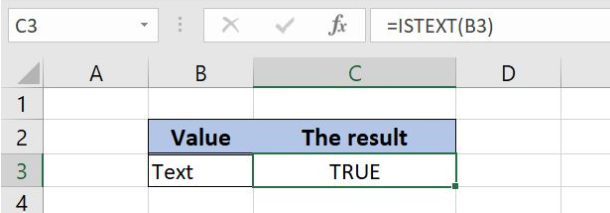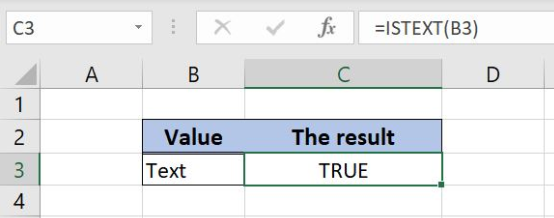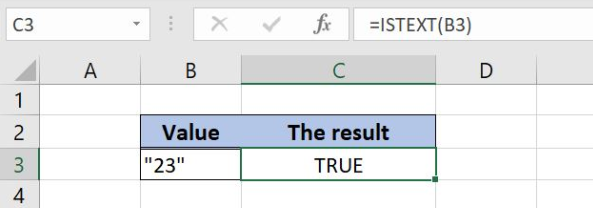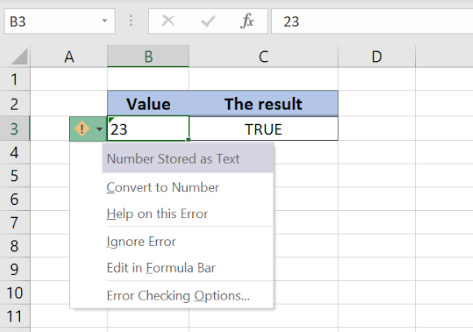Get instant live expert help with Excel or Google Sheets“My Excelchat expert helped me in less than 20 minutes, saving me what would have been 5 hours of work!”

#### Post your problem and you’ll get Expert help in seconds.

Your message must be at least 40 characters
Our professional Expert are available now. Your privacy is guaranteed.

# A Guide to the ISTEXT Function in Excel

Excel allows a user to check if a value is text, by using the ISTEXT function. This step by step tutorial will assist all levels of Excel users in checking if a value or a value of a cell is text.Figure 1. The result of the ISTEXT function

## Syntax of the ISTEXT Formula

The generic formula for the ISTEXT function is:

`=ISTEXT(value)`

The parameter of the ISTEXT function is:

• value – a value or a cell which we want to check if it is text

If a selected value is text, the function will return TRUE as a result. Otherwise, it will return FALSE.

## Check If Cells Value is Text by the ISTEXT function

In our example, we want to check if the value from the cell B3 is text. In B3, we put “Text” as the value.

The formula is:

`=ISTEXT(B3)`

The parameter value is the cell B3, while the result is in the cell C3.

To apply the ISTEXT function, we need to follow these steps:

• Select cell C3 and click on it
• Insert the formula: `=ISTEXT(B3)`
• Press enterFigure 2. Using the ISTEXT function to check if the value in the cell is text

The value in B3 is text, so the function returns Boolean TRUE as a result in the cell C3.

If we put a number in the cell B3, the function will return FALSE. If we put a number under double quotations, the function considers it as text and returns TRUE:Figure 3. Using the ISTEXT function to check if the value in the cell is text

## Notes

There is a specific case when using the ISTEXT function – checking numbers stored as text. If the number is stored as a text, the ISTEXT will return TRUE:Figure 4. The ISTEXT checking the number stored as text

In the cell B3, we put 3, but the cell is formatted as text. Because of that, the ISTEXT returns TRUE as a result.

Most of the time, the problem you will need to solve will be more complex than a simple application of a formula or function. If you want to save hours of research and frustration, try our live Excelchat service! Our Excel Experts are available 24/7 to answer any Excel question you may have. We guarantee a connection within 30 seconds and a customized solution within 20 minutes.

### Did this post not answer your question? Get a solution from connecting with the expert.Another blog reader asked this question today on Excelchat:## Subscribe to Excelchat.coAnother blog reader asked this question today on Excelchat: